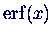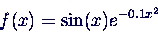# Numerical Applications

Maple

1. Write a function to convert degrees into radians. Test it with the following values:
30, 45, 90, 180, 720, 1 degree

2. Find the first three derivatives of the following functions:and integrate the results again.

3. Compute the taylor series of the functionup to the order.
Plotand the approximating taylor ploynoms.

Matlab

1. Write a function to convert degrees into radians. Test it with the following values:
30, 45, 90, 180, 720, 1 degree

2. Create a vector that contains the values of the functionin the interval -10 .. 10, and plot it. Create a plot of the subintervall -1 .. 1.

Repeat it with the functionand have a closer look at the region around 0.

3. The phobos image contains three neighbouring columns, which are brighter than their surroundings. Find them and correct them by scaling them with an approprate factor.
Create and plot a large image made up of the following four images:
-
phobos with bad spots,
-